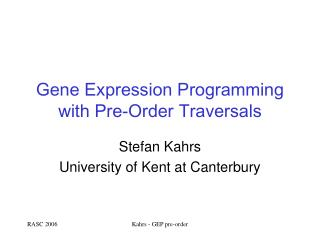# Gene Expression Programming with Pre-Order Traversals - PowerPoint PPT PresentationDownload PresentationGene Expression Programming with Pre-Order Traversals

Gene Expression Programming with Pre-Order TraversalsDownload Presentation## Gene Expression Programming with Pre-Order Traversals

- - - - - - - - - - - - - - - - - - - - - - - - - - - E N D - - - - - - - - - - - - - - - - - - - - - - - - - - -
##### Presentation Transcript

1. Gene Expression Programming with Pre-Order Traversals Stefan Kahrs University of Kent at Canterbury Kahrs - GEP pre-order

2. Overview • intro • gene expression programming and grammar evolution • breeding by crossover, meaning • change to pre-order traversal • a problem with that • ... and its solution Kahrs - GEP pre-order

3. breed fitness criterion mutate select Genetic Programming population of programs Kahrs - GEP pre-order

4. GEP and GE • separate genotype and phenotype • genetic information (determining an individual) is given as the list of nodes of the syntax tree in level-order (GEP) orpre-order (GE) • from this a syntax tree is recovered • the list can have additional node symbols that do not contribute to the tree (allows for mutation) Kahrs - GEP pre-order

5. Why GEP/GE? • separation of genotype/phenotype allows the manipulation of program individuals (optimisation) without interfering with breeding • avoids program bloat, program sizes are bounded Kahrs - GEP pre-order

6. + sin * x 2 + x y Example, level-order traversal +, sin, *, x, 2, +, x, y Kahrs - GEP pre-order

7. + sin + * x y x 2 Example, pre-order traversal +, sin, *, x, 2, +, x, y Kahrs - GEP pre-order

8. Cross-over breeding • take two individuals A and B • throw a dice to determine a crossover pointk • create a new individual whose first k symbols are the first k symbols of A, and its remaining ones are all but the first k symbols of B Kahrs - GEP pre-order

9. In Pictures but it does not quite work that way, in general Kahrs - GEP pre-order

10. Arity • we can extend the notion of arity from symbols to sequences of symbols arity() = 1 arity(s·f) = arity(s)-1+arity(f), if arity(s)>0 arity(s·f) = 0, otherwise • explanation: top-down tree traversal, placing f in the unfinished tree closes an open place and opens arity(f) new ones Kahrs - GEP pre-order

11. Example • take our previous sequence +, sin, *, x, 2, +, x, y • take the initial segment of the first 5 symbols, i.e. +, sin, *, x, 2 • its arity is 1 • this means: if we draw the incomplete tree of this segment there is 1 hole left to be filled with a subtree • this incomplete tree segment would be part of the offspring if this individual fathered with crossover point 5 Kahrs - GEP pre-order

12. Procreation with different arity at crossover point Kahrs - GEP pre-order

13. + + sin * sin * x 2 sin + 2 * x y 1 x y Trees with arity 1 and 2 at crossover point 5 Kahrs - GEP pre-order

14. Offspring, father left tree The subtree that has been placed in the gap is not a subtree of the mother, though it is composed from nodes from the mother + sin * x 2 * x y Kahrs - GEP pre-order

15. So... • if the arities are the same at cross-over point then subtrees of the mother are preserved • otherwise, mum is atomised and reassembled • making the fresh subtrees fairly random • problems: • asymmetric contribution in procreation • level of randomness that is hard to control Kahrs - GEP pre-order

16. A solution • replace level-order traversal with pre-order traversal (which GE uses anyway) • why? in pre-order traversal subtrees occur as consecutive subsequences • notion of arity (of sequences) is unchanged! Kahrs - GEP pre-order

17. + + sin * sin * x 2 sin + 2 * x y 1 x y Crossover at 6, different arities Kahrs - GEP pre-order

18. * 1 y Crossover at 6, different arities + the subtrees pasted into the tree are either from the mother, or the dormant part of the mother (here: the z) sin * x 2 + z Kahrs - GEP pre-order

19. A problem • how do we generate the initial population? • with level-order traversal we place (random) non-nullary symbols at the beginning of the sequence, nullary symbols at the end – the result are balanced trees • if we do this with pre-order traversal we get lop-sided trees (more lists than trees) Kahrs - GEP pre-order

20. Alternatively • we can meddle with the probabilities to prevent lop-sidedness, i.e. same probability for opening/closing a branch • problem: this gives us microbes (very small trees) and these buggers breed as if the end of the world is nigh Kahrs - GEP pre-order

21. Solution • level-order traversal works well at the beginning, pre-order traversal works well subsequently, so: why not use them this way! • create the initial population by reading a sequence as a level-order traversal • then traverse those trees pre-orderly, and overwrite the genome • from then on, use pre-order only Kahrs - GEP pre-order